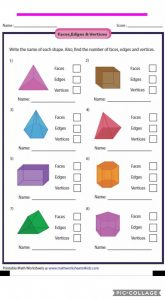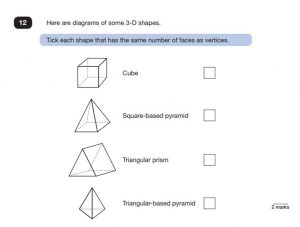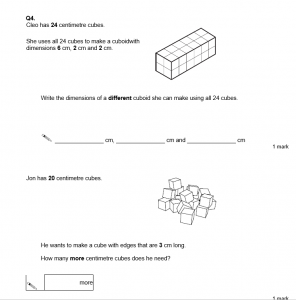### 3D shape homeworkVincent

1) Triangular-based pyramid f=4 e=6 v=4
2) Cube f=6 e=12 v=8
3) Pentagonal pyramid f=6 e=10 v=6
4) Square-based pyramid f=5 e=8 v=5
5) Cuboid f=6 e=12 v=8
6) Hexagonal prism f=8 e=18 v=12
7) Triangular prism f=5 e=9 v=6
8) Pentagonal prism f=7 e=15 v=10
1)Square-based pyramid and Triangular- based pyramid.
1)6cm, 4cm and 1cm.
2) 27 more cubes

Ankai

1)triangular based pyramid 2)Cube 3)pentagonal based pyramid
Faces:4 Faces:6 Faces:6
Edges:6 Edges:12 Edges:10
Vertices:4 Vertices:8 Vertices:6

4)square based pyramid 5)Cuboid
Faces:5 Faces:6
Edges:8 Edges:12
Vertices:5 Vertices:8

Ok that all for today, imma go live my life for a bitMrs Bell

Year 6 Teacher

Hi, I am Mrs Bell. With me there is never a dull moment! It has been said that if you are looking for me in school you will hear me before you see me (as I am really loud). I love musicals and I see everyday as a performance. I love to sing, act, and make other people laugh!